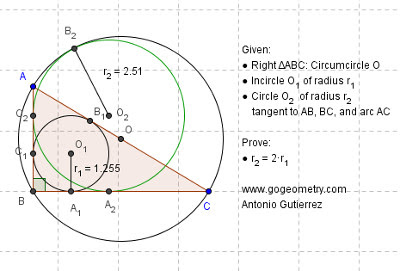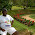## Thursday, September 10, 2015

### Dynamic Geometry Problem 1148: Right Triangle, Circle, Incircle, Circumcircle, Tangent, Radius. GeoGebra, Tablets

Geometry Problem. GeoGebra, HTML5 Animation for iPad and Nexus.
Post your solution in the comments box below.

Level: Mathematics Education, High School, Honors Geometry, College.

Click here to see the dynamic diagram of problem 1148.
Click here to see the static diagram of problem 1148.#### 14 comments:

1.From triangle BA2C2, we can write: A1O!/BC2 =A1A2/BA2 or (r2 -r1)/r2 =r1/r2 which yields, r2 -r1 =r1 or
r2 =2*r1

1.Ạjit

The statement " A1O1/BC2 =A1A2/BA2 " ís true if points A2, O1 , C2 are collinear .
Not clear how points A2, O1 , C2 are collinear.
I note that your solution don't use the given data " Circle O2 tangent to arc AC" . Please explain.

Peter Tran

2.Dear Ajit

Did u assume that O1 is the centre of square BA2O2C2? If so how?

Also your solution does not seem to use the given data that circles O and O2 are internally tangent. Could u kindly clarify?

2.Algebra solution:
Let O2C2= x
And AB= C, BC= a and AC= b
We have BO2= x.sqrt(2)
Let ∠(OBC) = θ
In triangle OBO2 we have OO2^2=OB^2+O2B^2-2. OB.O2B. cos(45- θ)……..(1)
Replace sin(θ)= c/b , cos(θ)= a/b
OB= b/2 in (1) we have
OO2^2= b^2/4+2.x^2-x.(a+c)….. (2)
Since circle (O2, x) tangent to circle (O, b/2) so
OO2= b/2-x or OO2^2= b^2/4+x^2-b.x….. ( 3)
Compare (2) and (3) we will have x= a+c- b= 2.r1

3.If BC=a; AB=b; C= diameter of circle O=R=C/2; r1= In-radium; C2O2 = r2 ; b=√(c^2-a^2 )
If r2 = 2 r1, centrum of r2 is O2 on the square C2BA2O2; We know that r1=(a.b)/(a+b+c) ;
Now we have just to try to show that r2 in o2 is tangent to circle O, if so the point B2O2O are necessary 1/2congruent.
We pose R= r2 +O2O in which O2O is the hypotenuse of triangle ODO2 and O2O =√(OD^2+〖DO2〗^2 ) in
Which CD= a/2-2r1 and DO2 =2r1 –b/2; so O2O=[ (a/2-2r1)2+(2r1-b/2)]1/2 in which
2r1=2a√(c^2-a^2 )/a+c+√(c^2-a^2 )
We can show that for every C and every a (a<C) that we introduce in the last formula we have
R= C/2=2r1 +O2 O and therefore r2=2r1 is tangent to A2,B2,C2
Replay

4.My thinking was: BC2B1A2 is a square of which BB1 & A2C2 are diagonals that intersect in O1 given that BC2=BA2=r2 (tangents to O2 from B). Hence etc

5.For convenience of typing denote in-radius r1 by r, radius r2 of circle O2 by s
In the usual notation, it is well-known that 2R = b, r = (a + c – b)/2
Referred to lines BC, BA as axes , O = (a/2 , c/2), O1 = (r, r), O2 = (s, s)
Due to tangency OO2 = (b/2) – s
Using distance formula:
[(b/2) – s)]^2 = IOO2I^2 = [(a/2) - s]^2 + [(c/2 - s]^2 which simplifies to
s^2 = ( c + a – b) s = 2 r s
Hence s = 2 r

6.Note first that OO2B2 are collinear as well as BO1O2.

In centre of Tr. ABC = r1 = 1/2(b+c-a) and r = b/2 where r is the circumradius of Right Tr. ABC. Draw OM perpendicular to O2A2.

Consider right Tr. MOO2.

OO2 = r - r2, MO2 = r2 - c/2, MO = a/2 - r2
Now applying Pythagoras
(b/2 - r2)^2 = (a - r2)^2 + (r2 - c/2)^2. Simplifying ;
r2^2 - (a+c-b)r2 + (c^2 + a^2 - b^2)/4 = 0

The 3rd term in the above equation is zero since ABC is a right Tr.
So r2 = a+c-b = 2r1

Sumith Peiris
Moratuwa
Sri Lanka

7.Note - the general formula for the inradius r1 = area / s where s is the semi perimeter.

So r1 = bc/s. Multiplying numerator and denominator by a+c-b and simplifying we arrive at r1 = 1/2 (a+c-b) which is the formula I used above though computing in a much simpler way using the fact that < B = 90

8.Dear Antonio - do u have a simpler method to prove this? With an auxiliary construction?

Rgds

Sumith

9.Dear Sumith,
My solution is like yours

1. Poncelet theorem a + c - b = 2r1
2. Tangent circles: line of centers: b/2 – r2
3. Triangle Mid-segment theorem: a/2, b/2, c/2
4. Pythagorean theorem a^2+b^2 = c^2

Probably we need to find a new auxiliary construction for another elegant solution.

Thanks
Antonio

10.I'm sure there is. Let's try.

11.O1 is the center of the square BA2O2C2. So O2A2 = 2 O1A1 etc

1.How is this Praveen?